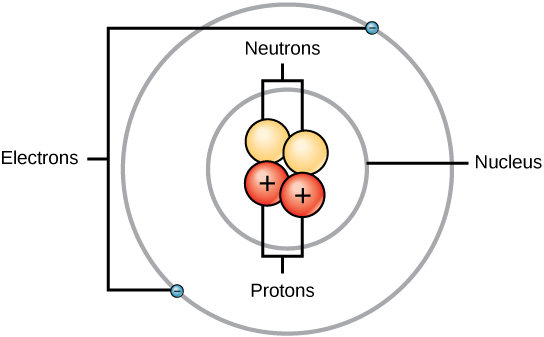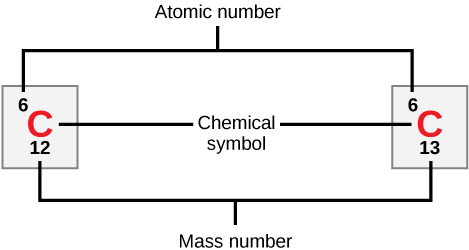## Atoms

### Learning Outcomes

• Draw a diagram of an atom, according to current scientific understanding

## Structure of the Atom

To understand how elements come together, we must first discuss the smallest component or building block of an element, the atom. An atom is the smallest unit of matter that retains all of the chemical properties of an element. For example, one gold atom has all of the properties of gold in that it is a solid metal at room temperature. A gold coin is simply a very large number of gold atoms molded into the shape of a coin and containing small amounts of other elements known as impurities. Gold atoms cannot be broken down into anything smaller while still retaining the properties of gold.

An atom is composed of two regions: the nucleus, which is in the center of the atom and contains protons and neutrons, and the outermost region of the atom which holds its electrons in orbit around the nucleus, as illustrated in Figure 1. Atoms contain protons, electrons, and neutrons, among other subatomic particles. The only exception is hydrogen (H), which is made of one proton and one electron with no neutrons.Figure 1. Elements, such as helium, depicted here, are made up of atoms. Atoms are made up of protons and neutrons located within the nucleus, with electrons in orbitals surrounding the nucleus.

Protons and neutrons have approximately the same mass, about 1.67 × 10–24 grams. Scientists arbitrarily define this amount of mass as one atomic mass unit (amu) or one Dalton, as shown in Table 1. Although similar in mass, protons and neutrons differ in their electric charge. A proton is positively charged whereas a neutron is uncharged. Therefore, the number of neutrons in an atom contributes significantly to its mass, but not to its charge. Electrons are much smaller in mass than protons, weighing only 9.11 × 10–28 grams, or about 1/1800 of an atomic mass unit. Hence, they do not contribute much to an element’s overall atomic mass. Therefore, when considering atomic mass, it is customary to ignore the mass of any electrons and calculate the atom’s mass based on the number of protons and neutrons alone. Although not significant contributors to mass, electrons do contribute greatly to the atom’s charge, as each electron has a negative charge equal to the positive charge of a proton. In uncharged, neutral atoms, the number of electrons orbiting the nucleus is equal to the number of protons inside the nucleus. In these atoms, the positive and negative charges cancel each other out, leading to an atom with no net charge.

Accounting for the sizes of protons, neutrons, and electrons, most of the volume of an atom—greater than 99 percent—is, in fact, empty space. With all this empty space, one might ask why so-called solid objects do not just pass through one another. The reason they do not is that the electrons that surround all atoms are negatively charged and negative charges repel each other.

Table 1. Protons, Neutrons, and Electrons
Charge Mass (amu) Location
Proton +1 1 nucleus
Neutron 0 1 nucleus
Electron –1 0 orbitals

## Atomic Number and Mass

Atoms of each element contain a characteristic number of protons and electrons. The number of protons determines an element’s atomic number and is used to distinguish one element from another. The number of neutrons is variable, resulting in isotopes, which are different forms of the same atom that vary only in the number of neutrons they possess. Together, the number of protons and the number of neutrons determine an element’s mass number, as illustrated in Figure 2. Note that the small contribution of mass from electrons is disregarded in calculating the mass number. This approximation of mass can be used to easily calculate how many neutrons an element has by simply subtracting the number of protons from the mass number. Since an element’s isotopes will have slightly different mass numbers, scientists also determine the atomic mass, which is the calculated mean of the mass number for its naturally occurring isotopes. Often, the resulting number contains a fraction. For example, the atomic mass of chlorine (Cl) is 35.45 because chlorine is composed of several isotopes, some (the majority) with atomic mass 35 (17 protons and 18 neutrons) and some with atomic mass 37 (17 protons and 20 neutrons).

### Practice Question

Carbon has an atomic number of six, and two stable isotopes with mass numbers of twelve and thirteen, respectively. Its atomic mass is 12.11.Figure 2. Carbon-12 and carbon-13

How many neutrons do carbon-12 and carbon-13 have, respectively?

## Contribute!

Did you have an idea for improving this content? We’d love your input.